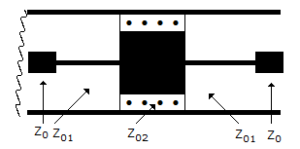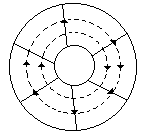# Multiple Choice Questions and Answers on Microwave Communication

Q1. A cavity resonator is

a) a hollow metallic enclosure
b) a hollow enclosure having magnetic material as its walls
c) a hollow enclosure having dielectric material as its walls
d) either (b) or (c)

Q2. If antenna diameter is increased four times, the maximum range is increased by a factor of

a) √2
b) 2
c) 4
d) 0.2

Q3. Assertion (A): Gunn diode is a transferred electron device.

Reason (R): A Gunn oscillator uses the phenomenon of transferred electron effect.

a) Both A and R are correct and R is correct explanation of A
b) Both A and R are correct but R is not correct explanation of A
c) A is correct but R is wrong
d) A is wrong but R is correct

Q4. Atomic and molecular resonance is observed in many substances

a) at microwave frequencies
b) at low frequencies
c) at frequencies used in AM broadcast
d) at both (b) and (c)

Q5. The component in the given figure isa) attenuator
b) T type low pass filter
c) T type high pass filter
d) phase shifter

Q6. The semiconductor diode which can be used in switching circuit in microwave range is

a) PIN diode
b) Varactor diode
c) Tunnel diode
d) Gunn diode

Q7. In the given figure the reflected current wave after first reflection isa)  0.18 A
b) -0.18 A
c) 0.3 A
d) -0.3 A

Q8. A line has an attenuation of 0.054 Np/m. The attenuation in decibels is

a) 4.7 dB/m
b) 0.47 dB/m
c) 0.54 dB/m
d) 5.4 dB/m

Q9. If a line is open circuited Zin = Z0 tanh (gl).

a) True
b) False

Q10. A reflex klystron oscillator uses

a) one cavity resonator
b) two cavity resonators
c) three cavity resonators
d) none of the above

Q11. A coaxial RF cable has a characteristic impedance of 50 Ω and C equal to 40 pF/m. The inductance is

a) 1 μ H/m
b) 10 μ H/m
c) 0.1 μ H/m
d) 0.01 μ H/m

Q12. To couple a coaxial line to a parallel wire line it is best to use

a) slotted line
b) balun
c) directional coupler
d) λ/4 transformer

Q13. In a multicavity klystron amplifier the signal to be amplified develops an ac voltage of signal frequency across the gap in buncher cavity

a) True
b) False

Q14. If VSWR is infinite, the transmission line is terminated in

a) short circuit
b) complex impedance
c) open circuit
d) either (a) or (c)

Q15. In a circular waveguide TE21 mode has lowest cutoff frequency

a) True
b) False

Q16. The number of TV channels which can be accommodated in a spectrum of 300 MHz is about

a) 5
b) 10
c) 50
d) 200

Q17. The noise figure of multicavity klystron amplifier is very low

a) True
b) False

Q18. Assertion (A): The velocity of electromagnetic waves on overhead lines and coaxial cables is the same.

Reason (R): Free space has an intrinsic impedance of 377 ohms.

a) Both A and R are correct and R is correct explanation of A
b) Both A and R are correct but R is not correct explanation of A
c) A is correct but R is wrong
d) A is wrong but R is correct

Q19. In the given figure the E and H lines in a coaxial cablea) The radial lines are E lines and circular lines are H lines
b) Radial lines are H lines and circular lines are E lines
c) The directions of E and H lines are wrong
d) Radial lines may be E and H lines depending an direction of current

Q20. Roughly the time required for microwave cooking as compared to conventional cooking is

Q21. If the minimum range of a radar is to be doubled, the peak power has to be increased by a factor of

a) 2
b) 4
c) 8
d) 16

Q22. Which mode has the lowest cut off frequency in circular wave guides?

a) TE01
b) TE11
c) TE20
d) TE21

Q23. A wave Em cos (bx – ωt) is a backward wave.

a) True
b) False

Q24. Assertion (A): Klystron amplifiers use one or more intermediate cavities in addition to buncher and catcher cavity.

Reason (R): When one or more intermediate cavities are used the bandwidth can be increased.

a) Both A and R are correct and R is correct explanation of A
b) Both A and R are correct but R is not correct explanation of A
c) A is correct but R is wrong
d) A is wrong but R is correct

Q25. The reflection coefficient on a line is 0.2 ∠45°. The SWR is

a) 0.8
b) 1.1
c) 1.2
d) 1.5

Q26. In a circular waveguide the dominant mode is

a) TE01
b) TE11
c) TE20
d) TE21

Q27. In a klystron amplifier the input cavity is called

a) buncher
b) catcher
c) Pierce gun
d) collector

Q28. A loss less line of characteristic impedance Z0 is terminated in pure reactance of –jZ0 value. VSWR is

a) 10
b) 2
c) 1
d) infinity

Q29. The velocity factor of a transmission line depends on

a) temperature
b) skin effect
c) relative permittivity of dielectric
d) none of the above

Q30. Microwave resonators are used in

a) microwave oscillators
b) microwave narrow band amplifier
c) microwave frequency metres
d) all of the above

Q31. Assertion (A): TWT uses a focussing mechanism to prevent the electron beam from spreading.

Reason (R): In a TWT the electron beam has to travel a much longer distance than in klystron.

a) Both A and R are correct and R is correct explanation of A
b) Both A and R are correct but R is not correct explanation of A
c) A is correct but R is wrong
d) A is wrong but R is correct

Q32. In a TWT the amplitude of resultant wave travelling down the helix

a) increases exponentially
b) increases linearly
c) decreases exponentially
d) is almost constant

Q33. Which of the following is not a travelling wave?

a) e = Em sin (βx – ωt)
b) e = Em cos (βx – ωt)
c) e = Em sin (ωt – βx)
d) e = Em sin (βx)

Q34. Both Impatt and Trapatt devices use avalanche effect

a) True
b) False

Q35. In a directional coupler

a) isolation (dB) equals coupling plus directivity
b) coupling (dB) equals isolation plus directivity
c) directivity (dB) equals isolation plus coupling
d) isolation (dB) equals (coupling) (directivity)

Q36. As the frequency is increased, the charging MVAR in a cable

a) decreases
b) increases
c) remain the same
d) decreases or remains the scheme

Q37. Loss angle of a good quality cable is about

a) 1°
b) 30°
c) 70°
d) 90°

Q38. In a three cavity klystron amplifier, the oscillations are excited in

a) input cavity
b) output cavity
c) intermediate cavity
d) both (a) and (b)

Q39. Which of the following lines is non-radiating?

a) Open two wire
b) Coaxial
c) Both
d) None of the above

Q40. Assertion (A): The impedance of a matched load is equal to characteristic impedance of line.

Reason (R): A matched termination absorbs all the power incident on it.

a) Both A and R are correct and R is correct explanation of A
b) Both A and R are correct but R is not correct explanation of A
c) A is correct but R is wrong
d) A is wrong but R is correct

Q41. Skin effect is more pronounced at high frequencies.

a) True
b) False

Q42. Assertion (A): The phenomenon of differential mobility is called transferred electron effect.

Reason (R): GaAs exhibits transferred electron effect.

a) Both A and R are correct and R is correct explanation of A
b) Both A and R are correct but R is not correct explanation of A
c) A is correct but R is wrong
d) A is wrong but R is correct

Q43. Which of the following devices uses a helix?

a) Klystron amplifier
b) Klystron oscillator
c) TWT
d) Both (a) and (b)

Q44. Which of the following parameters is negligible in transmission lines?

a) R
b) L
c) C
d) G

Q45. The width of a radio beam from a 1 m diameter parabolic antenna at 10 GHz is about

a) 100°
b) 50°
c) 5°
d) 1°

Q46. The diagram to show distance time history of electrons in klystron amplifier is called

a) apple gate diagram
b) asynchronous diagram
c) bunching diagram
d) velocity modulation diagram

Q47. A quarter wave line open circuited at far end behaves as

a) inductance
b) L and C in parallel
c) capacitance
d) L and C in series

Q48. The directivity in a receiving antenna

1. increases the intercept area in forward direction
2. reduces the noise picked up from other sources
3. provides a means of discriminating against undesired signals originating in directions other than in which the desired transmitter lies.

Which of the above statements are correct?

a) 1 only
b) 1 and 2 only
c) 1, 2 and 3
d) 2 and 3 only

Q49. The bunching action which occurs in multicavity klystron amplifier can be represented by Applegate diagram

a) True
b) False

Q50. The cut off wavelength in circular waveguide

a) guide diameter
b) square of guide diameter
c) cube of guide diameter
d) square root of guide diameter

Q51. The directive gain of a transmitting antenna is proportional to

a) its cross-sectional area
b) square of cross-sectional area
c) square root of cross-sectional area
d) cube root of cross-sectional area

Q52. In a loss line RL < Z0, then

a) Reflection coefficient is zero
b) Reflection coefficient is ∞
c) Reflection coefficient is negative
d) Reflection coefficient is positive

Q53. If a line having Z0 = 300 ∠ 0 W is open circuited at far end, VSWR is

a) 0
b) 1
c) ∞
d) 2

Q54. If f is the frequency of electromagnetic wave, fc is cutoff frequency, then in a rectangular waveguide

a) attenuation is low when f > fc
b) attenuation is low when f < fc
c) attenuation is high when f < fc
d) either (b) or (c)